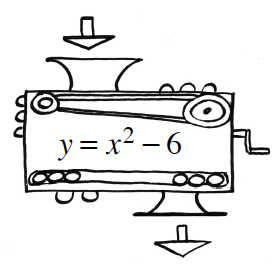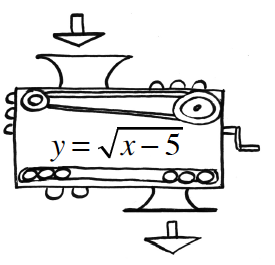### Home > CCA > Chapter 1 > Lesson 1.1.1 > Problem1-4

1-4.Angelica is working with function machines. She has the two machines shown at right. She wants to put them in order so that the output of the first machine becomes the input of the second. She wants to use a beginning input of 6.

1. In what order must she put the machines to get a final output of $5$?

Substitute $6$ for each $x$ in the first function.

$\textit{y}=\sqrt{6-5}$

$y=1$

Substitute the answer of the first
equation for $x$ in the second equation.

$y=1^2−6$

$y=−5$

INCORRECT! Try Again!

Substitute $6$ into the $2$nd equation first!

$y=6^2−6$

$y=30$

Substitute the answer of the $2$nd
equation for $x$ in the 1st equation.

$\textit{y} = \sqrt{30 - 5}$

$y=5$

CORRECT!

2. Is it possible for her to find an input that will get a final output of $-5$? If so, show how she could do that. If not, explain why not.

See above (Try 1).